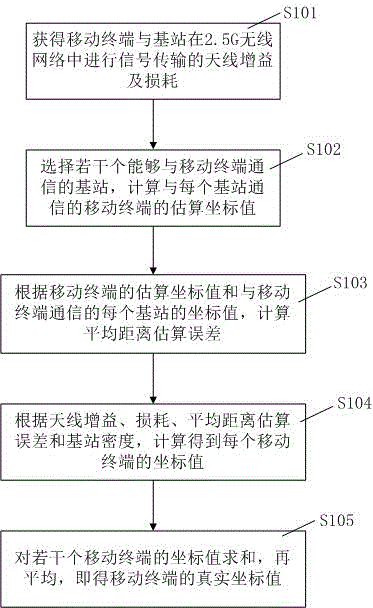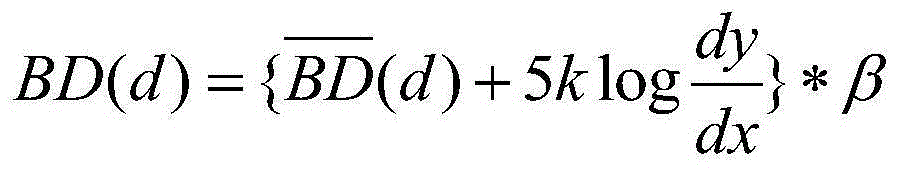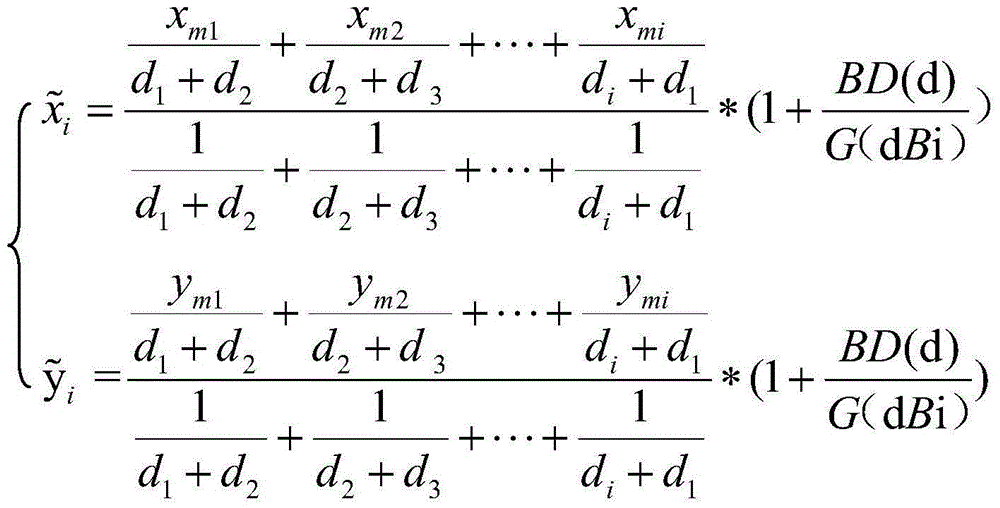# Positioning algorithm based on signal gain and loss of 2.5G wireless network

## A wireless network signal and positioning algorithm technology, applied in the field of positioning algorithms based on 2.5G wireless network signal gain and loss, can solve the problems of low positioning sensitivity and low positioning accuracy.

Inactive Publication Date: 2015-11-25
CHENGDU GOLDENWAY ELECTRONICS
5 Cites 4 Cited by

## AI-Extracted Technical Summary

### Problems solved by technology

 Aiming at the above-mentioned problems existing in the prior art, the purpose of the present invention is to provide a positioning algorithm based on the signal gain and loss of 2...
View more

## Abstract

The invention discloses a positioning algorithm based on signal gain and loss of a 2.5G wireless network. The algorithm comprises the following steps: calculating antenna gain and signal loss of mobile terminals and base stations during signal transmission in the 2.5G wireless network; obtaining an estimated coordinate value of a mobile terminal communicating with each base station; through the estimated coordinate values and coordinate value information of each base station communicating with the mobile terminals, obtaining an average distance estimated error through calculation; according to the antenna gain, the loss, the average distance estimated error and the density of the base stations, obtaining coordinate values of the plurality of mobile terminals through calculation; and solving a sum of the coordinate values of the plurality of mobile terminals, and then performing averaging to obtain real coordinate values of the mobile terminals so that the mobile terminals are positioned under the condition of the signal loss of the 2.5G wireless network. The algorithm provided by the invention is based on full consideration of the loss of signals in a transmission process, is more accurate in positioning, is suitable for outdoor application, and also has a quite good positioning effect in indoor application.

Application Domain

Position fixationWireless communication

Technology Topic

VIT signalsTransfer procedure +5

## Image

•••## Examples

• Experimental program(1)

### Example Embodiment

 In order to make the objectives, technical solutions and advantages of the present invention clearer, the technical solutions of the present invention will be described clearly and completely in conjunction with specific embodiments of the present invention and the corresponding drawings.
 See figure 1 , figure 1 To adopt the flow chart S100 of the positioning algorithm based on the 2.5G wireless network signal gains and losses of the present invention; the flow chart S100 includes steps S101 to S105;
 In step S101, obtain the antenna gain and loss of the mobile terminal and the base station for signal transmission in the 2.5G wireless network; high-gain directional antennas must be used in the 2.5G wireless network. The narrower the antenna main lobe width, the higher the gain. Under a certain power, the higher the gain, the larger the radiation range;
 According to an embodiment of the present application, in the signal transmission process between the mobile terminal and the base station, first, the antenna gain of the signal in the 2.5G wireless network transmission is calculated. The antenna gain G(dBi) can be calculated by the following formula (1):
 G(dBi)=10lg{50000/(2θ3dB,E×2θ3dB,H)}(1)
 Among them, (2θ3dB,E) and (2θ3dB,H) are the lobe widths of the antenna on the horizontal and vertical planes respectively, 50000 is the empirical data calculated in 2.5G wireless network; (2θ3dB,E) is the antenna on the horizontal plane The lobe width on the antenna can be 45, 60 or 90; (2θ3dB, H) is the lobe width of the antenna in the vertical plane, which can be 48, 33, 15 or 8;
 According to an embodiment of the present application, in a 2.5G wireless network, (2θ3dB, E) is 45 and (2θ3dB, H) is 48. According to the formula G(dBi)=10lg(50000/45*48), it is obtained The gain of the directional antenna.
 Since the signal is transmitted between the mobile terminal and the base station, a certain loss will be generated due to the influence of weather or obstacles, the following formula (2) is used to calculate the loss BD(d) caused by the signal in the transmission process:
 B D ( d ) = { B D ‾ ( d ) + 5 k l o g d y d x } * β - - - ( 2 )
 Among them, BD(d) is the path loss after signal transmission through distance d; Is the path loss of signal transmission after a unit distance; k is the path interference factor; 5 is the constant value of the signal in the 2.5G wireless network; dx is the receiving power of the 2.5G wireless network, and dy is the transmitting power of the 2.5G wireless network; β Correct the constant value for 2.5G wireless network to 1.342.
 In step S102, select a number of base stations that can communicate with mobile terminals, and calculate the estimated coordinate value with each base communication mobile terminal;
 According to an embodiment of the present application, the mobile terminal selects i base stations from all base stations that can communicate with it, estimates the approximate distance between the mobile terminal and each selected base station, and then calculates the distance value according to each estimate , Using the weighted centroid algorithm to calculate the estimated coordinate value of the mobile terminal communicating with each selected base station Calculated using weighted centroid algorithm The coordinate method can be calculated using the following formulas (3) and (4):
 x ~ i = x m 1 d 1 + d 2 + x m 2 d 2 + d 3 + ... + x m i d i + d 1 1 d 1 + d 2 + 1 d 2 + d 3 + ... + 1 d i + d 1 * ( 1 + B D ( d ) G ( d B i ) ) - - - ( 3 )
 y ~ i = y m 1 d 1 + d 2 + y m 2 d 2 + d 3 + ... + y m i d i + d 1 1 d 1 + d 2 + 1 d 2 + d 3 + ... + 1 d i + d 1 * ( 1 + B D ( d ) G ( d B i ) ) - - - ( 4 )
 among them, The estimated coordinate value of the mobile terminal communicating with each base station selected for the mobile terminal; d i Is the estimated distance between the mobile terminal and the i-th base station; (x mi , Y mi ) Is the coordinate value of the i-th base station.
 In step S103, the estimated coordinate value of the mobile terminal and the coordinate value information of each base station communicating with the mobile terminal are used to calculate the average distance estimation error between the mobile terminal and the base station;
 According to an embodiment of the present application, the average distance estimation error between the mobile terminal and the base station is It can be calculated by the following formula (5):
 ϵ ‾ = X i = 1 n ( x ~ i - x m i ) 2 + ( y ~ i - y m i ) 2 N , ( i = 1 , 2 ... N ) - - - ( 5 )
 among them, Is the average distance estimation error; N is the number of times the algorithm is executed.
 In step S104, the coordinate value of each mobile terminal is calculated according to the antenna gain, loss, average distance estimation error and base station density;
 According to an embodiment of the present application, the coordinate value of a mobile terminal communicating with a certain base station can be calculated by the following formulas (6) and (7):
 X i = G ( d B i ) - B D ( d ) ϵ ‾ * D A + x m i - - - ( 6 ) Y i = G ( d B i ) - B D ( d ) ϵ ~ * D A + y m i - - - ( 7 )
 Among them, G(dBi) is the antenna gain; BD(d) is the signal loss during transmission; Is the average distance estimation error; DA is the base station density; (x mi , Y mi ) Is the coordinate value of the i-th base station;
 Among them, the base station density can be calculated by the following formula (8):
 D A = ( A Q A Q + N Q ) · 100 %
 Among them, AQ is the number of selected base stations; NQ is the number of unselected base stations that can communicate with the mobile terminal.
 In step S105, the coordinate values ​​of several mobile terminals are summed, and then averaged to obtain the real coordinate values ​​of the mobile terminal;
 According to an embodiment of the present application, the communication coverage areas of a number of selected base stations capable of communicating with mobile terminals in a 2.5G wireless network overlap with each other. When the mobile terminal receives signal packets from N different base stations, respectively Calculate the coordinates of the mobile terminal communicating with each base station, and then sum up the coordinates of several mobile terminals obtained, and then average them to obtain the real coordinates of the mobile terminal, which realizes the mobile terminal's real coordinates in the 2.5G wireless network. For positioning, the following formula (8) can be used to calculate the real coordinates (X dst , Y dst ):
 ( X d s t , Y d s t ) = ( 1 N X i = 1 N X i , 1 N X i = 1 N Y i ) , ( i = 1 , 2 ... N ) - - - ( 8 )
 Where (X dst , Y dst ) Is the real coordinate value of the mobile terminal; (X i , Y i ) Is the coordinate value of the mobile terminal communicating with each selected base station.
 The positioning algorithm of the present invention realizes the precise positioning of the mobile terminal in the 2.5G wireless network, changes the traditional positioning algorithm that does not consider the loss of the signal in the transmission process and the gain of the directional antenna, the positioning sensitivity is high, and it is suitable for For indoor and outdoor positioning, the application range is greatly improved.

## PUM## Description & Claims & Application Information

We can also present the details of the Description, Claims and Application information to help users get a comprehensive understanding of the technical details of the patent, such as background art, summary of invention, brief description of drawings, description of embodiments, and other original content. On the other hand, users can also determine the specific scope of protection of the technology through the list of claims; as well as understand the changes in the life cycle of the technology with the presentation of the patent timeline. Login to view more.
Who we serve
• R&D Engineer
• R&D Manager
• IP Professional
Why Eureka